# Section 4.2. Interference Effects on IEEE 802.11ab WLANs

### 4.2. Interference Effects on IEEE 802.11a/b WLANs

In this section, we consider the specific performance degradations to IEEE 802.11a/b systems resulting from UWB interference. The IEEE 802.11a and 802.11b standards are specifications for wireless local area networks. Devices conforming to the 802.11a standard transmit data using OFDM modulation in the unlicensed 5 GHz band (the so-called Unlicensed National Information Infrastructure, or U-NII). These devices are capable of transmitting data at up to 54 Mbps for distances up to 30 meters. The high operating frequency limits the penetration properties of such devices, and as a result their range is also restricted to short distances. In contrast, 802.11b WLAN systems (also known as Wi-Fi devices) transmit data using DSSS modulation in the 2.4 GHz radio band (known as the industrial, scientific, and medical [ISM] band). The lower operating frequency allows 802.11b devices to penetrate through walls and other obstructions to provide a range up to 100 meters for data rates as high as 11 Mbps. Both 802.11a and 802.11b use the Ethernet protocol and the Carrier Sense Multiple Access with Collision Avoidance (CSMA/CA) protocol for multiple-access channels.

Considering the UWB permitted region of operation from 3.1 GHz to 10.6 GHz, 802.11a technology is particularly affected as it lies in the main UWB transmission band, as shown in Figure 4-1. In contrast, the 802.11b band is not located in the main UWB transmission band, so these devices are affected somewhat less than 802.11a systems. The reason is that the UWB interference results from purely out-of-band interference for 802.11b systems. In order to compute and analyze the aggregate impact of multiple UWB devices on WLAN systems, we first need to model an 802.11a/b victim receiver (either a mobile station or an access point) in the presence of several UWB devices.

#### 4.2.1. Interference Model

We consider the situation where a WLAN node is surrounded by several UWB transmitters in a two-dimensional setting. Figure 4-2 shows the model scenario, where the victim receiver is placed at the center of two concentric circles.

##### Figure 4-2. The interference scenario: a WLAN node placed among UWB transmitters in communication with an access point.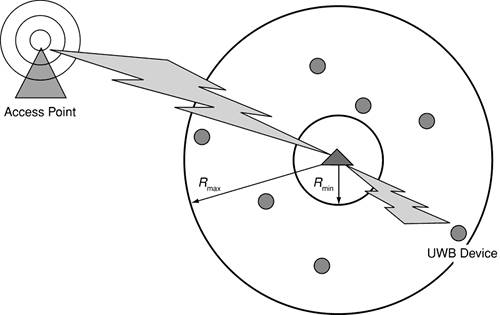The victim WLAN receiver is shown as a triangle in the center of the circles. The inner circle defines the boundary of a UWB-free zone; that is, no UWB transmitters are closer to the victim than Rmin. In between the inner and outer circles (given by Rmax), the UWB transmitters are distributed uniformly over the surface. All the interfering UWB devices are assumed to be transmitting with equal power. This power is given by the maximum PSD defined in the relevant frequency band by the FCC/ETSI emission mask, as well as the actually employed bandwidth of the victim system. Further, we assume that there is no co-channel interference; that is, there is no interference from other access points using the same frequency. In a likely indoor environment, this is a reasonable assumption.

Equations 4-1 through 4-3 provide the basis for our analysis of the possible UWB transmitter density around an 802.11a/b device. The mathematical derivations of these equations are presented in Appendix A. Equation 4-1 shows the UWB interference power that represents the total received power from all UWB devices around the victim receiver.

Equation 4-1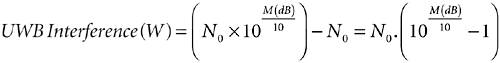where N0 is the thermal noise (that is, we consider there is no neighboring channel interference) and M is defined as the amount of degradation in the signal-to-interference ratio (SINR) that the system can support.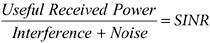UWB interference can also be defined as a function of UWB transmitter density:

Equation 4-2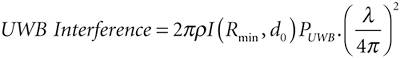where r is the UWB transmitter density in users per square meter; PUWB is the transmission power for each UWB device (fixed by the maximum of the emission mask); l is the wavelength; and I(Rmin, d0) is a constant depending on the environment through d0 (the breakpoint) and Rmin. Therefore, the maximum permissible UWB transmitter density r under the constraint that the SINR decreases not more than M dB can be calculated as

 The propagation loss breakpoint (d0) is a change in the slope of the propagation loss with distance at a radial distance from the transmitter. It is caused by the reflection of the transmitted signal by the ground. This multipath signal interferes with the direct path signal and usually occurs only in areas with clear line-of-sight and ground reflection paths.

Equation 4-3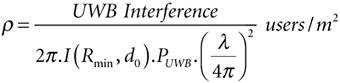Using these equations we can evaluate the permitted UWB density for various decreases in the SINR and estimate effects due to the introduction of UWB devices on IEEE 802.11a/b WLANs. The propagation loss formula used in all of the calculations in this section is the free space propagation loss and the inverse fourth-power law before and after the breakpoint value.

#### 4.2.2. WLAN Cell Coverage

The cell coverage is a main consideration of WLAN systems as it defines the maximum possible data rates as a function of the distance and, more directly, as a function of the SINR. In practice, we use the receiver sensitivity in order to define the cell radius such that we have a desired quality of service. The coverage range is evaluated with the maximum transmission power at the access point. WLAN manufacturers do not have any information about environments and therefore interference levels. For this reason, they introduce an implementation margin such that the system can support some fluctuation in the signal strength.

Examples of the fluctuation in signal strength are: fading due to the movement of people, penetration loss due to walls and so on, or an increase in the noise floor due to additive interference from spurious emitters in the receiver bandwidth. This margin guarantees (to some extent) that the received signal will always be greater than the minimum required at a certain distance for a given data rate.

Figure 4-3 illustrates the minimum signal level for each data rate according to the manufacturer (implementation margin plus receiver noise factor). Since the receiver's output noise is a combination of channel noise and the noise generated inside a receiver, the output signal-to-noise ratio (SNR) is usually less than the input SNR. The ratio of input to output SNR is defined as the noise figure or noise factor.

##### Figure 4-3. Minimum signal level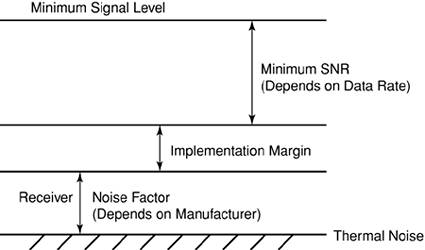##### 802.11a

The IEEE 802.11a standard splits the spectrum into three bands of four pilot channels per band; and different regions of the world allocate different amounts of spectrum. Indeed, three bands have been allocated for the United States, the lowest and the middle band have been allocated for Europe, and only the lowest band is available in Japan. Hence, there are 12 available channels in the United States, 8 in Europe, and only 4 in Japan. The IEEE 802.11a standard requires receivers to have a minimum sensitivity ranging from 82 dBm to 65 dBm, depending on the chosen and/or required data rate . Table 4-1 provides an overview of data rates based on minimum sensitivity and minimum SNR.

##### Table 4-1. 802.11a minimum signal level, data rate, and SNR

Data Rate (Mbps)

Minimum Signal Level (dBm)

Minimum SNR

6

82

5

9

81

8

12

79

10

18

77

13

24

74

16

36

70

19

48

66

22

54

65

25

From the standard described in ITU-R M.1450 , the minimum signal level (shown in Figure 4-3) is measured at the antenna connector with a 5 dB implementation margin and a 10 dB receiver noise factor. If we consider the United States and Europe, we have at least eight channels for indoor coverage. Therefore, because indoor cells are in general not very large, we can neglect channel interference due to other access points using the same frequency. Indeed, considering a typical building with dimensions 20 meters by 50 meters and N floors, one access point is sufficient for the coverage; however, two access points could be considered in order to take capacity into account. Therefore, with eight available channels, co-channel interference is not a problem even if we consider the interference from floors N 1 and N + 1. Signals from other floors will be largely attenuated as a result of the penetration losses, and hence the interference from other floors will be small even if the same frequency is used. Thus, the coverage is determined by a noise-limited environment.

In order to assess the effect of the UWB interference, we now compute a maximum distance fixed by the SNR. In other words, we compute the maximum radius such that we reach the minimum SNR (5 dB, from Table 4-1) at this distance. Typical coverage is obtained through the receiver sensitivity, but as described before, there are different margins according to manufacturers; therefore we get different minimum signal levels and thus different topologies. Figures 4-4 through 4-6 represent the relationship between SNR and distance, the achievable link data rate versus SNR, and finally the achievable link data rate versus distance between the user and the access point for an indoor environment using 802.11a WLAN.

##### Figure 4-4. SNR as a function of radius, with d0 = 5 m, for 802.11a WLAN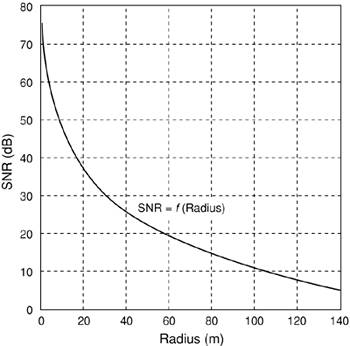##### Figure 4-5. Data rate as a function of SNR, for 802.11a WLAN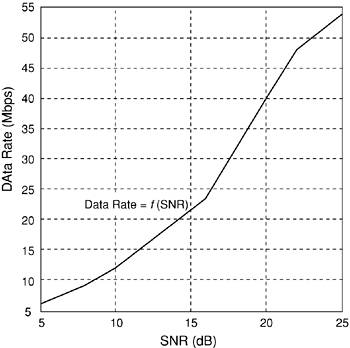##### Figure 4-6. Data rate as a function of radius, for 802.11a WLAN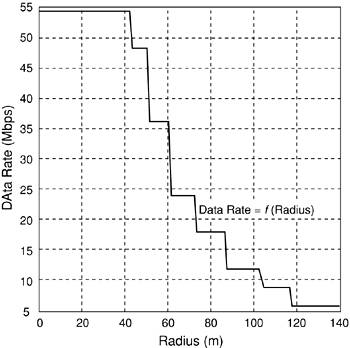Figure 4-4 shows that the useful signal decreases with an increase in the distance between the user and the access point. Therefore, the SNR decreases because the noise is constant and the signal is decreasing.

Devices utilizing 802.11a are required to support data rates up to 54 Mbps. As shown in Figure 4-5, a data rate of 54 Mbps can be achieved for 802.11a WLAN systems when the SNR is 25 dB. Figure 4-6 gives the coverage with maximum possible data rates as a function of the distance for 802.11a devices.

Figure 4-6 shows that the data rate of 54 Mbps is achievable when the distance between the user and an access point is less than 40 to 42 meters. When the user is located about 118 meters away from the access point, a 6 Mbps data rate is achievable with 802.11a systems.

##### 802.11b

Similar to 802.11a devices, coverage is an important factor for 802.11b systems also. However, there is no information in the IEEE standard  about minimum SNRs as a function of the data rates for such systems. So in general, the typical minimum sensitivities, ranging from 93 dBm to 84 dBm, depending on the chosen data rate (see Table 4-2) will be considered here for understanding the coverage in 802.11b devices.

##### Table 4-2. Data rate and receiver sensitivity

Data Rate (Mbps)

Receiver Sensitivity (dBm)

1

93

2

90

5.5

87

11

84

Figures 4-7 through 4-9 show the relationship between SNR and distance, the achievable link data rate versus SNR, and finally the achievable link data rate versus distance between the user and access point for an indoor environment using 802.11b WLAN.

##### Figure 4-7. SNR as a function of radius, for 802.11b WLAN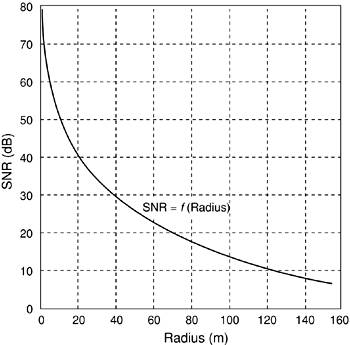##### Figure 4-8. SNR as a function of data rate, for 802.11b WLAN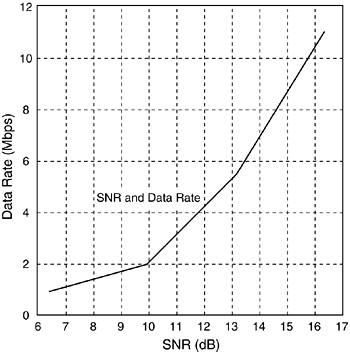##### Figure 4-9. Data rate and radius, for 802.11b WLAN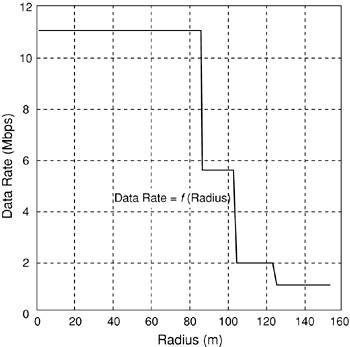Figure 4-7 shows that, as in the previous case for 802.11a, SNR decreases with an increased distance between the user and the access point. We compute the SNR for an indoor environment with transmit power equal to 100 mW. The propagation breakpoint value considered is 5 meters (d0 = 5 m). Therefore, from Table 4-2 we can estimate the SNR required for a given data rate, as shown in Figure 4-8.

Devices utilizing 802.11b are required to support speeds up to 11 Mbps. This depends strongly on the SNR. Therefore, from Figures 4-7 and 4-8, we can estimate the possible data rate as a function of distance, as shown in Figure 4-9.

Figure 4-9 demonstrates the coverage with possible data rates as a function of the distance. As shown, the highest data rate of 11 Mbps in 802.11b devices is achievable when the distance between the user and the access point is less than 80 to 85 meters. Therefore, when the user is located about 150 meters away from the access point, a 1 Mbps data rate is achievable with 802.11b devices.

#### 4.2.3. UWB Emission Limits in the IEEE 802.11a/b Band

As explained in Chapter 1, in both FCC and ETSI regulations, the frequency spectrum used for UWB communications devices is between 3.1 GHz and 10.6 GHz, with a maximum PSD of 41.3 dBm/MHz. The FCC distinguishes between two types of devices: indoor and outdoor. Indoor devices are allowed higher emissions than outdoor devices. For this reason, indoor devices must be capable of indoor operation only and are not allowed to be directed to the outside of a building.

##### 802.11a

802.11a devices use 300 MHz of bandwidth in the 5 GHz unlicensed band, and this bandwidth is divided into three distinct 100 MHz bands. With the use of the orthogonal frequency-division multiplexing (OFDM) modulation scheme, each band is split into four 20 MHz subbands, which give a total of twelve 20 MHz channels across the 300 MHz bandwidth. Therefore, the receiver bandwidth for an 802.11a device is 20 MHz. Because of the very wide bandwidth of UWB devices, it is assumed that the UWB spectrum is flat in the 802.11a device bandwidth; therefore, the UWB spectrum is considered to be like white noise. The UWB PSD in the 802.11a band for both the ETSI and the FCC is:

 White noise is characterized by its flat power spectral density, meaning that its power is constant for all frequencies.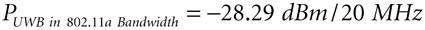##### 802.11b

In the 802.11b band, the PSD limits are 51.3 dBm/MHz for indoor and 63.3 dBm/MHz for outdoor applications. However, ETSI does not distinguish between outdoor and indoor applications but rather in terms of required reliability. This does not, however, affect the power spectral density levels (or EIRP), which are given by:

 EIRP represents effective isotropic radiated power, which is equivalent to the power of a signal transmitted from an omnidirectional (isotropic) antenna.

Equation 4-4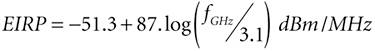Therefore, in the 802.11b band, we have an emission limit of 61 dBm/MHz. As was the case for the 802.11a computations, we consider the UWB signal to be white in the bandwidth of interest.

With the FCC Mask

PUWB in 802.11a Bandwidth = -37.87 dBm/22 MHz for indoor

PUWB in 802.11a Bandwidth = -47.87 dBm/22 MHz for outdoor

With the ETSI Mask

PUWB in 802.11a Bandwidth = -47.57 dBm/22 MHz for both indoor and outdoor

#### 4.2.4. Interference as a Function of UWB Transmitter Density

In order to find the interference as a function of UWB transmitter density, we consider a uniform distribution of UWB systems around the victim receiver taken from Rmin to infinity, as previously detailed in Section 4.2.1. In indoor environments, such as homes and offices, one expects to find high UWB transmitter densities. In fact, it is realistic to have a maximum of two active UWB devices in a surface area of 10 square meters. It is also important to emphasize that the number of active devices is considered to be substantially fewer than the number of physically present devices. Thus, we work with values from 0.01 to 0.2 users per square meter (equal to one to two users in 10 square meters) for both 802.11a and 802.11b cases.

##### 802.11a

From Equations 4-1 through 4-3, we obtain the results shown in Figures 4-10 through 4-12 for IEEE 802.11a as a function of Rmin and (active) UWB device densities.

##### Figure 4-10. Cumulative UWB interference at an 802.11a victim receiver with d0 = 10 m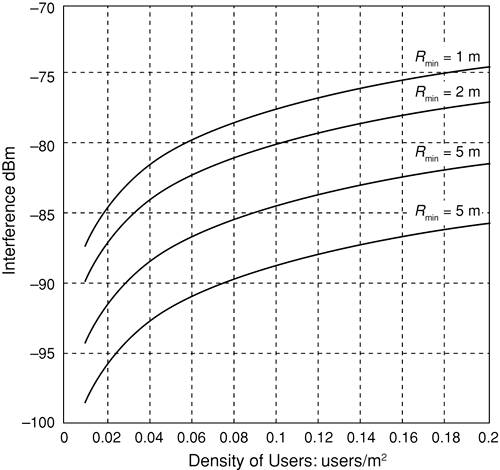##### Figure 4-11. SINR as a function of radius with d0 = 5 m and UWB interference, for 802.11a WLAN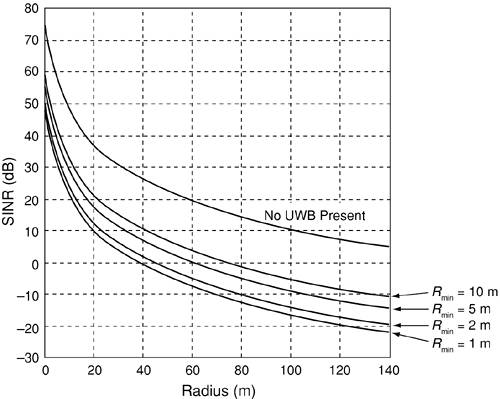##### Figure 4-12. Data rate as a function of radius with UWB interference, for 802.11a WLAN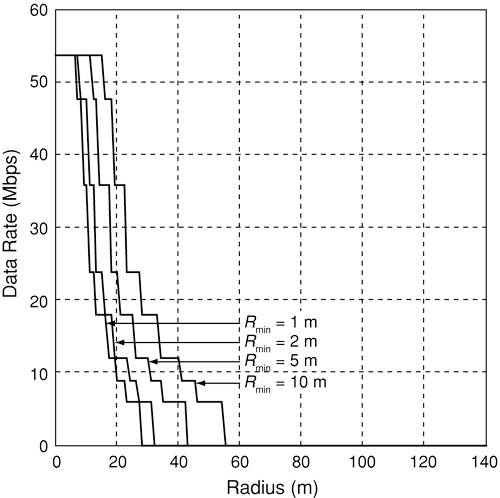As shown in Figure 4-10, the amount of UWB interference increases with the increase in the UWB density and at the same time the most damage is caused by the group of UWB devices 1 meter away from the WLAN device. Therefore, with the introduction of UWB devices around the victim receiver, we have:

Equation 4.5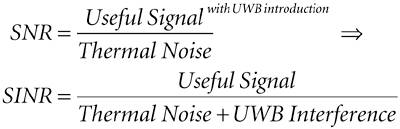In Figures 4-11 and 4-12, we consider the effects due to the introduction of UWB devices on the victim SINR with UWB density r = 0.2 users/m2 (that is, two users in 10 square meters) and examine the degradation of the performance. In Figure 4-12 we observe both modifications in the coverage and data rate.

As shown in Figure 4-11, the SINR decreases as a function of the radius. The reason is that with any increase in radius, the useful signal decreases, whereas the noise plus UWB interference remains constant; therefore, the SINR decreases in the same manner. We observe that for certain values of the radius, the SINR is negative; this is due to the fact that the noise plus interference becomes more important than the useful signal.

Figure 4-12 gives the coverage with possible data rates as a function of the distance. With the introduction of UWB devices, we observe a modification for both coverage and possible data rates. For example, with Rmin = 1 meter, the 802.11a coverage is lowered by more than a factor of four (compared to the case without UWB introduction, as shown in Figure 4-6).

Comparing Figure 4-6 with Figure 4-12 shows that with the use of either the FCC or ETSI power spectral densities, serious disturbance of an 802.11a system can result for a typical UWB transmitter density (that is, r = 0.2 users per square meter). Since 802.11a uses a physical carrier-sensing protocol besides the virtual carrier sensing, it is even possible that strong UWB interference may appear to a node as though another user is transmitting, thereby causing the node to hold back its transmission. In the case of very strong interference, this could cause an additional drop in performance.

##### 802.11b

Similar to the 802.11a case in the previous subsection, we use Equation 4-1 to obtain the results shown in Figures 4-13 through Figure 4-18 for IEEE 802.11b as a function of Rmin and (active) UWB device densities for both FCC and ETSI masks.

##### Figure 4-13. UWB interference as a function of density and Rmin with d0 = 5 m, for 802.11b WLAN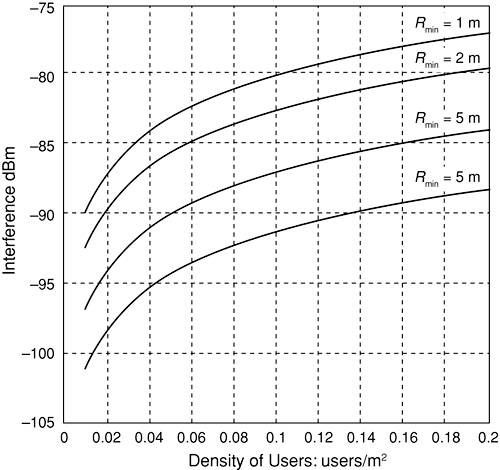##### Figure 4-14. SINR as a function of radius with UWB interference, for 802.11b WLAN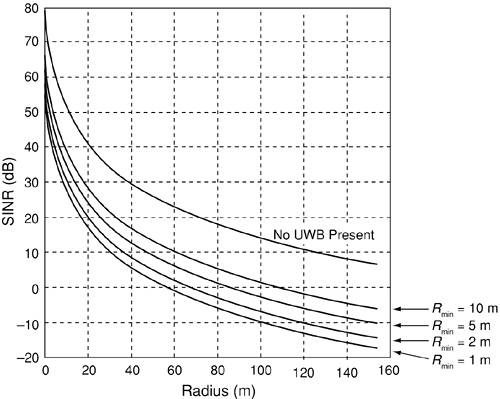##### Figure 4-15. Data rate and radius with UWB interference, for 802.11b WLAN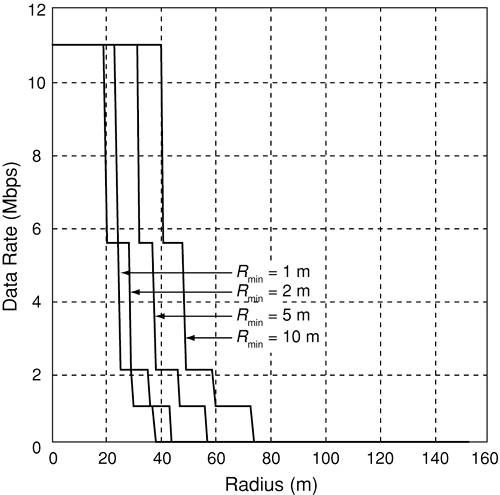##### Figure 4-16. UWB interference as a function of density and Rmin, for 802.11b WLAN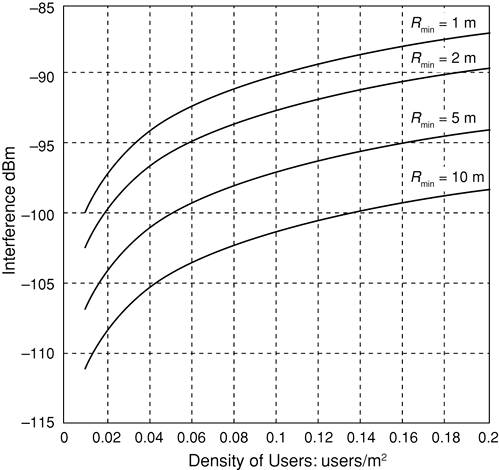##### Figure 4-17. SINR as a function of radius, with d0 = 5 m, for 802.11b WLAN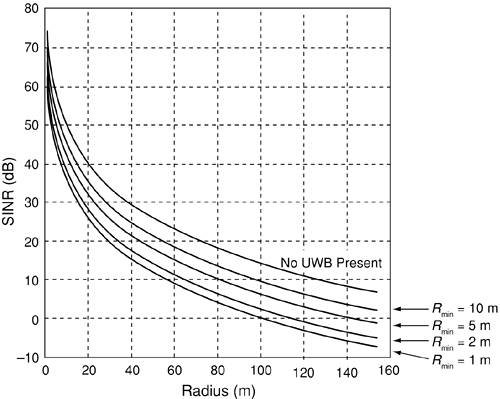##### Figure 4-18. Data rate and radius with UWB interference, with d0 = 5 m, for 802.11b WLAN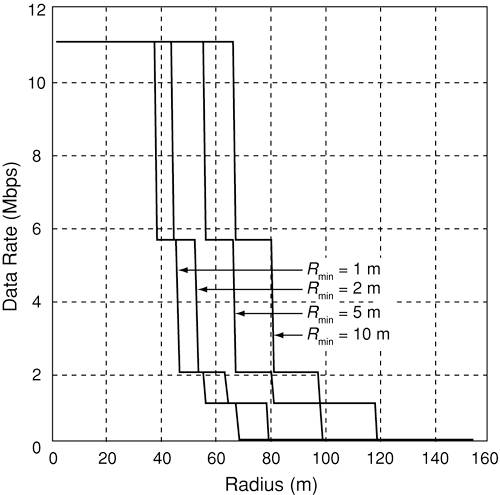With FCC Mask: Indoor = 37.87 dBm/22 MHz

After introducing UWB emissions to the indoor channel with a density of 0.2 users per square meter, we compute the SNR with transmit power of 100 mW for various distances between the victim receiver and the first UWB device (Rmin).

Next we concentrate on the 802.11b coverage, with possible data rates as a function of the distance, as shown in Figure 4-16. With the introduction of UWB devices, we observe a modification in both the coverage and the possible data rates.

With a UWB density of 0.2 users per square meter, we compute the SNR for an indoor environment with transmit power equal to 100 mW for different Rmin (minimum distance between the victim receiver and the first UWB device).

With ETSI Mask: 47.57 dBm/22 MHz

Figure 4-18 gives the coverage with possible data rate as a function of the distance. With the introduction of UWB devices, we observe a modification in both the coverage and the possible data rate in 802.11b devices.

#### 4.2.5. UWB Density Permitted for 1 dB Degradation in the SNR

If the WLAN can tolerate a 1 dB degradation in the SNR, from Equation 4-1 the permitted received UWB interference at the victim node is such that:

Equation 4-6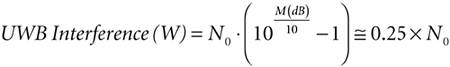Therefore, based on the calculated UWB interference, the UWB density from Equation 4-3 will be:

Equation 4-7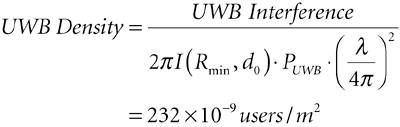where I(Rmin, d0) is computed with Rmin = 1 meter and d0 = 5 meters and PUWB is the UWB emission limit fixed by ETSI and the FCC in 802.11a bandwidth.

##### 802.11a

With N0 in Equation 4-6 equal to 101 dBm (the thermal noise in the 802.11a receiver bandwidth of 20 MHz), a 1 dB degradation in the SINR corresponds to about a 20 percent decrease in the received SINR. So the UWB interference, W, and the UWB transmitter density (active users) can be estimated from Equations 4-1 and 4-3, respectively.

802.11a WLAN

UWB Interference (dBm)

107

UWB Density (users/m2)

232 x 109

This analysis shows that for a 1 dB decrease in the SNR, the UWB density permitted by the system is really too low for any practical usage. Therefore, we conclude that the density found is too small to consider realistic UWB densities for cohabitation between UWB and 802.11a devices. Consequently, UWB deployment will be nearly impossible in an 802.11a network area if we can only tolerate 1 dB degradation in the SINR and further measures to improve performance are not available.

##### 802.11b

With N0 in Equation 4-6 equal to 100.5 dBm (the thermal noise in the 802.11b receiver bandwidth of 22 MHz), a 1 dB degradation in the SINR corresponds to about a 20 percent decrease in the received SINR. As in the previous case, we can estimate the UWB interference (W) and the UWB transmitter density (active users) with the FCC outdoor mask from Equations 4-1 and 4-3, respectively.

802.11b WLAN

UWB Interference (dBm)

106.6

UWB Density (users/m2)

2 x 104

As expected, 802.11b devices have more tolerance of interference from UWB transmitters. The main reason is that UWB interference is considered to be out-of-band interference for 802.11b devices, as opposed to in-band interference for 802.11a systems.

#### 4.2.6. Minimum Distance with One UWB Transmitter

In order to represent the effect of UWB interference to 802.11a and 802.11b devices, we consider only one UWB transmitter and estimate the necessary minimum separation distance to degrade the signal by no more than M dB. (See Figure 4-19.)

##### Figure 4-19. Minimum distance between UWB device and victim receiver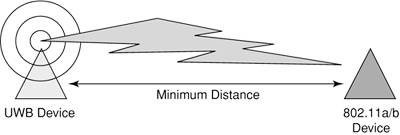If we consider an M dB degradation in the SNR, the maximum UWB interference level will be the following:

Equation 4-8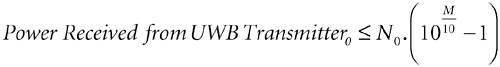The power at a UWB receiver, considering free-space propagation loss before the breakpoint value, d0, and a fourth-power loss law after the breakpoint, can be modeled as:

Equation 4-9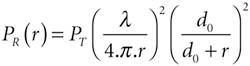where PR is the received power, PT is the transmit power, l is the wavelength, and r is the distance between the UWB transmitter and the victim receiver. A typical value for an indoor environment is d0 = 5 meters for links between an access point/terminal station and UWB devices/terminal station. By using Equations 4-8 and 4-9, we can obtain the minimum separation distance r between a UWB device and an 802.11a or 802.11b device.

Equation 4-10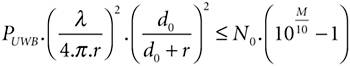Considering the current FCC indoor UWB emission limits, the separation distance can be calculated as follows.

1 dB Degradation

802.11a

802.11b

FCC

11.5 m

9.27 m

ETSI

11.5 m

4.53 m

These calculations show that if we consider UWB deployment, especially in office environments, the minimum distance between a UWB device and a victim receiver does not seem to permit coexistence with 802.11a or 802.11b systems without potentially serious performance degradation.

#### 4.2.7. Maximum Possible UWB Transmission Power for Realistic UWB Densities

We have seen that the use of either the FCC or the ETSI limits can lead to serious disturbances of an 802.11a or 802.11b system. We could in theory shape our spectrum to greatly reduce that interference, so that an 802.11a or 802.11b access point and UWB transmitters can operate in close vicinity such that we have a realistic UWB density around the victim receiver. In order to do this, we consider a given UWB density, taken here as 0.2 users per square meter, and we assume the maximum permissible UWB interference power such that:

Equation 4-11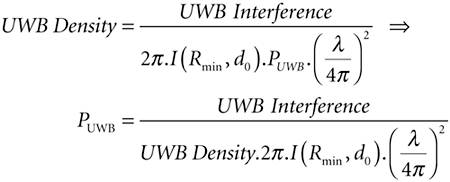Therefore, from Equation 4-11, for a 1 dB decrease in the SNR permitted by the system, we obtain the following results for 802.11a and 802.11b systems.

802.11a

802.11b

UWB EIRP for 1 dB Degradation

122.57 dBm/MHz

80.5 dBm/MHz

Thus, taking into account the actual emission limit of 41.3 dBm/MHz, coexistence between UWB and 802.11a devices could be realized if the emission mask is at least 72 dB below the current FCC or ETSI limit. This could potentially be achieved using a multiband approach in the UWB transmitters.

FCC and ETSI regulations allow UWB devices to operate within a 7.5 GHz-wide transmission spectrum between 3.1 GHz and 10.6 GHz. As explained in Chapter 1, the multiband technology divides this spectrum into several bands that can be added or dropped based on interference from other systems. The UWB Multi-Band Coalition, which represents members and supporters such as Intel, Staccato Communications, Time Domain, Philips, and General Atomics, and others, tries to unify existing UWB technologies to enable coexistence and interoperability. Their UWB multiband concept is based on the principle of partitioning the 7,500 MHz of unlicensed UWB spectrum into several smaller bands. Thus we can imagine that when the system detects the presence of an 802.11a system, for example, it can avoid the use of the bands centered around 5.15 GHz to 5.85 GHz.

This same feature can also be used to provide for different spectrum allocations in the rest of the world. The bands that share the spectrum with extremely sensitive systems can be avoided. Therefore, these subbands can be used with different techniques, such as frequency hopping where the frequency used for the modulation goes up and down between subcarriers available in 4.1 GHz to 10.6 GHz or simply the use of all channels that yield the total data rate from each subband.

#### 4.2.8. Comparison of Possible Data Rates for 802.11a and 802.11b with UWB Interference

The 802.11a and 802.11b standards each define a different physical layer. The 802.11b radios transmit at 2.4 GHz and send data up to 11 Mbps using DSSS modulation, whereas the 802.11a radios transmit at 5 GHz and send data up to 54 Mbps using OFDM modulation. The superior performance of 802.11a offers excellent support for applications that require high data rates, but the higher operating frequency equates to shorter range. The 802.11a standard offers more nonoverlapping channels per access point: eight versus three for 802.11b. These systems will need to operate with UWB devices present; therefore it's interesting to compare effects of UWB interference to both the possible data rates and coverage.

##### Without UWB Interference

In the absence of UWB interference, 802.11a has a performance advantage (see Figure 4-20). The figure shows that 802.11a delivers a greater data rate than 802.11b at the same distance, but the maximum coverage is less important in the absence of UWB interference.

##### Figure 4-20. Comparison of 802.11a and 802.11b data rates in the absence of UWB interference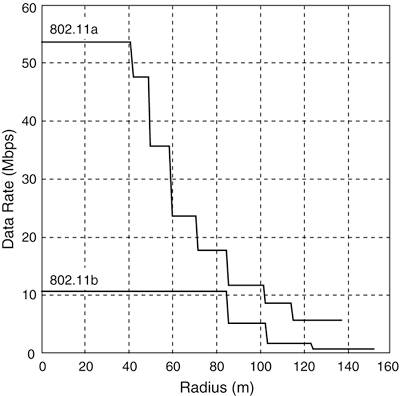##### With UWB Interference

In order to estimate the effects of UWB interference, we consider a UWB density of 0.2 users per square meter around the victim WLAN receiver and a minimum distance Rmin = 1 meter with the FCC and ETSI masks. (See Figures 4-21 and 4-22.)

##### Figure 4-21. Data rate versus radius, with the FCC mask, for UWB interference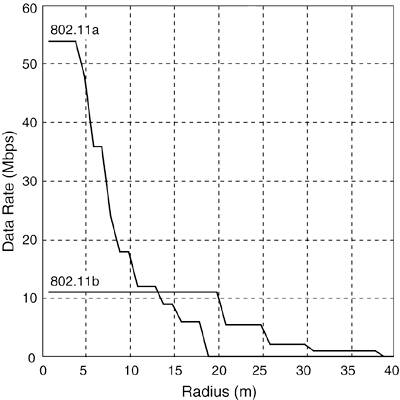##### Figure 4-22. Data rate versus radius, with the ETSI mask, for UWB interference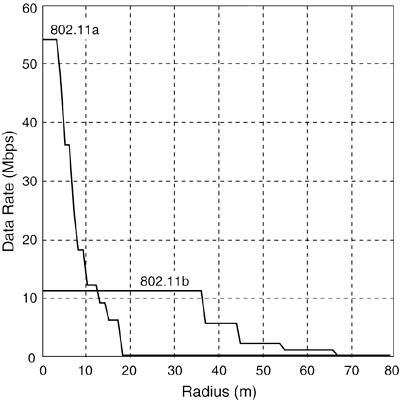We have seen that we can have harmful UWB interference to 802.11a; indeed, the coverage is divided by more than seven, whereas we have a factor of four for 802.11b's coverage.

With ETSI considerations, the UWB emission limit is about 10 dB less than with the FCC mask. Therefore, 802.11b's coverage is divided by only two, whereas we have no differences with FCC regulation for 802.11a's coverage due to the fact that FCC and ETSI emissions limits are the same in the 5 GHz band.

From Figures 4-21 and 4-22, we can see that the coverage of 802.11a is clearly more limited by the interference. Both systems can still reach their maximum rates at shorter distances. Although, if the data rate is the criterion, 802.11a would be a better choice for short distances. On the other hand, if range is the criterion, 802.11b performs better, especially under the ETSI regulations.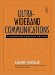Ultra-Wideband Communications: Fundamentals and Applications
ISBN: 0131463268
EAN: 2147483647
Year: 2005
Pages: 93

flylib.com © 2008-2017.
If you may any questions please contact us: flylib@qtcs.net